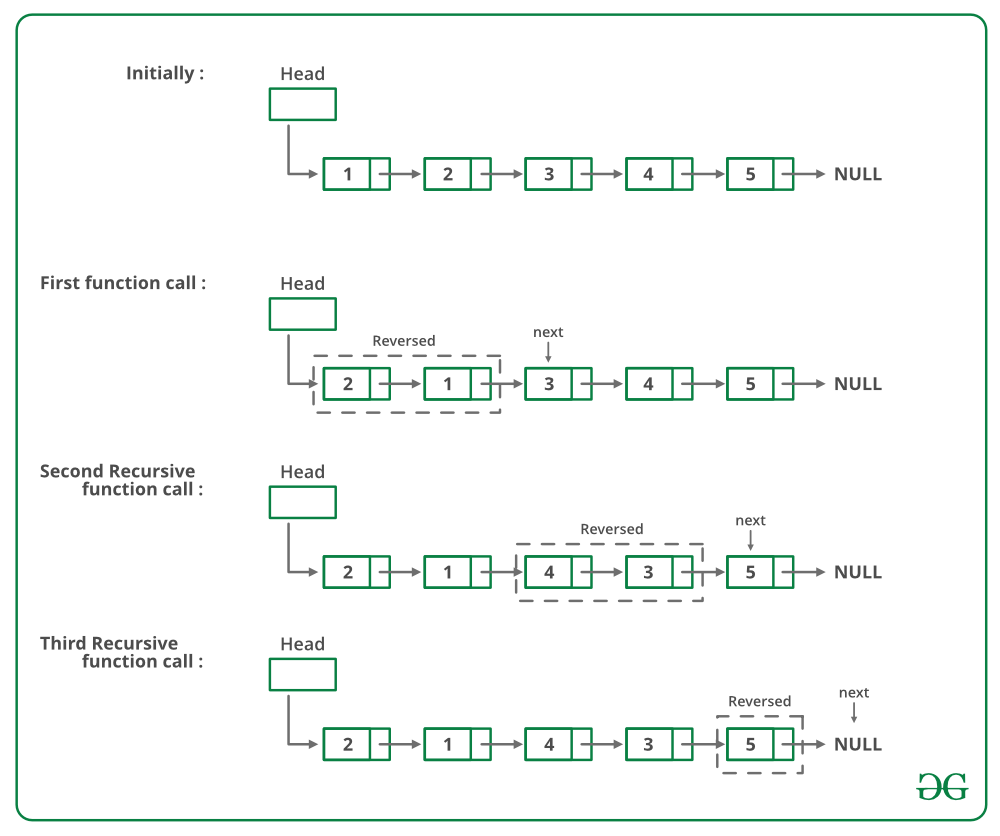GeeksforGeeks App
Open AppBrowser
Continue

# Javascript Program For Pairwise Swapping Elements Of A Given Linked List

Given a singly linked list, write a function to swap elements pairwise.

```Input: 1->2->3->4->5->6->NULL
Output: 2->1->4->3->6->5->NULL

Input: 1->2->3->4->5->NULL
Output: 2->1->4->3->5->NULL

Input: 1->NULL
Output: 1->NULL```

For example, if the linked list is 1->2->3->4->5 then the function should change it to 2->1->4->3->5, and if the linked list is then the function should change it to.

METHOD 1 (Iterative):
Start from the head node and traverse the list. While traversing swap data of each node with its next node’s data.
Below is the implementation of the above approach:

## Javascript

 ``

Output:

```Linked list before calling pairWiseSwap()
1 2 3 4 5
2 1 4 3 5 ```

Time complexity: O(n), as we are traversing over the linked list of size N using a while loop.

Auxiliary Space: O(1), as we are not using any extra space.

METHOD 2 (Recursive):
If there are 2 or more than 2 nodes in Linked List then swap the first two nodes and recursively call for rest of the list.
Below image is a dry run of the above approach:Below is the implementation of the above approach:

## Javascript

 ``

Time complexity: O(n), as we are traversing over the linked list of size N using recursion.

Auxiliary Space: O(1), as we are not using any extra space.
The solution provided there swaps data of nodes. If data contains many fields, there will be many swap operations. See this for an implementation that changes links rather than swapping data.

Please refer complete article on Pairwise swap elements of a given linked list for more details!

My Personal Notes arrow_drop_up